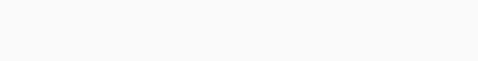# CountVectorizer to Extract Features from Text in Python

In order to use textual data for predictive modeling, the text data requires special preparation before you can start using it.

However, there are usually two steps performed on the textual data to get it prepared for ML tasks.

• Tokenization – The text must be parsed to extract certain words.
• Vectorization – Once the words are extracted,  they are encoded with integer or floating-point values to use as input for a machine-learning algorithm.

The scikit-learn library in python offers us tools to implement both tokenization and vectorization (feature extraction) on our textual data.

In this article, we see the use and implementation of one such tool called CountVectorizer.

Importing libraries, the CountVectorizer is in the sklearn.feature_extraction.text module.

```import pandas as pd
from sklearn.feature_extraction.text import CountVectorizer```

Let’s consider a simple text and implement the CountVectorizer.

```vectorizer = CountVectorizer()

text = ['CodeSpeedy Technology Private Limited is an Information technology company.']
print(text)

# tokenization
vectorizer.fit(text)
print(vectorizer.vocabulary_)```
`['CodeSpeedy Technology Private Limited is an Information technology company.']`

Here, we have first initialized the CountVectorizer() as vectorizer. Then taking a simple text, we apply fit() on the same.

For instance, the vectorizer.vocabulary_ gives us a dictionary in which each term is given an index (token) based on the starting letter of each word.

`{'codespeedy': 1, 'technology': 7, 'private': 6, 'limited': 5, 'is': 4, 'an': 0, 'information': 3, 'company': 2}`

```# vectorization
vector = vectorizer.transform(text)
print(vector)
print(vector.toarray())```

The vecotorizer.transform() on the text gives the occurrence of each word in the text.
For example, here the (0, 7) represents the word “technology” and the value 2 is the frequency of the word in the text.

```  (0, 0)	1
(0, 1)	1
(0, 2)	1
(0, 3)	1
(0, 4)	1
(0, 5)	1
(0, 6)	1
(0, 7)	2
[[1 1 1 1 1 1 1 2]]```

Also, one can read more about the parameters and attributes of CountVectorizer() here.

In conclusion, let’s make this info ready for any machine learning task.

```df = pd.DataFrame(data = vector.toarray(), columns = vectorizer.get_feature_names())
print(df)```